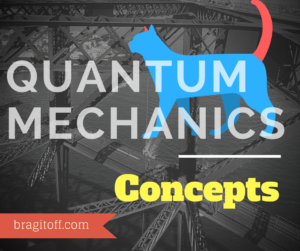# Harmonic Oscillator – Relativistic Correction

In this post, I will use the stationary(time-independent) first order perturbation theory, to find out the relativistic correction to the Energy of the nth state of a Harmonic Oscillator.In order to find out the relativistic correction to the Energy, we would need to use relativistic relations.
The relativistic Kinetic Energy is given as:$T= \sqrt{p^2c^2+m^2c^4}-mc^2$
The first term in the above equation is the total energy of a relativistic particle and the second term is the rest mass energy of a particle. So we get the Kinetic energy by subtracting those.$\implies T=mc^2\sqrt{1+\frac{p^2c^2}{m^2c^4}}-mc^2$

Now, using the binomial expansion on the Kinetic energy we can write it as:$T=mc^2(1+\frac{1}{2}\frac{p^2c^2}{m^2c^4}-\frac{1}{8}\left(\frac{p^2c^2}{m^2c^4}\right)^2+....)-mc^2$

Note: Binomial Expansion:$(1+x)^n=1+nx+n(n-1) \frac{x^2}{2} +...$$\implies T=(mc^2+\frac{p^2}{2m}-\frac{p^4}{8m^3c^4}+....)-mc^2$

If we ignore the rest of the higher order terms and take  only the first three terms of the Binomial expansion, then$\implies T=\frac{p^2}{2m}-\frac{p^4}{8m^3c^2}$

We can now see that the Kinetic Energy is actually modified and not just$\frac{p^2}{2m}$ as in the classical case. Since the second term would be very small due to$c^2$ in the denominator, we can take it as a perturbation, and use the time-independent perturbation theory to find out the correction to the energy levels.

Let perturbation,$H'=-\frac{p^4}{8m^3c^2}$

Then the first order energy correction to the nth level is given as:$E_n^1=\left<\psi_n\right|H'\left|\psi_n\right>$$\implies E_n^1=-\frac{1}{8m^3c^2}\left<\psi_n\right|p^4\left|\psi_n\right>$

From Schrodinger’s Equation:$\frac{p^2}{2m}\left|\psi_n\right>+V\left|\psi_n\right>=E_n\left|\psi_n\right>$$\implies p^2\left|\psi_n\right>=2m(E_n-V)\left|\psi_n\right>$

Using the above relation,$\implies E_n^1=-\frac{1}{8m^3c^2}\left<\psi_n\right|\left(2m(E_n-V)\right)^2\left|\psi_n\right>$$\implies E_n^1=-\frac{1}{2mc^2}\left<\psi_n\right|\left(E_n^2+V^2-2E_nV\right)\left|\psi_n\right>$$\implies E_n^1=-\frac{1}{2mc^2}\left(E_n^2+\left-2E_n\left\right)$

From Virial Theroem for Harmonic Oscillator, we know that the expectation value of V:$\left=\frac{E_n}{2}$$\implies E_n^1=-\frac{1}{2mc^2}\left(E_n^2+\left-E_n^2\right)$$\implies \boxed{ E_n^1=-\frac{1}{2mc^2}\left }$

So it all boils down to finding the expectation value of$V^2$.

To do that we would need to use the following relations, for a Harmonic Oscillator:$V=\frac{1}{2}m\omega^2 x^2$

and$x=\sqrt{\frac{\hbar}{2m\omega}}(\hat{a}+\hat{a}^\dagger)$

where$\hat{a}$ and$\hat{a}^\dagger$ are annihilation(lowering) and creation(raising) operators respectively.

Substituting the above value of$x$ in the expression for$V$,$V=\frac{1}{2}m\omega^2\frac{\hbar}{2m\omega}(a+a^\dagger)^2$$\implies V^2=\frac{1}{4}m^2\omega^4\left(\frac{\hbar}{2m\omega}\right)^2(\hat{a}+\hat{a}^\dagger)^4$$\implies V^2=\frac{\hbar^2\omega^2}{16}(\hat{a}+\hat{a}^\dagger)^4$

Now you might remember the following relations for the operators$\hat{a}$ and$\hat{a}^\dagger$,$\hat{a}\left|n\right>=\sqrt{n}\left|n-1\right>$$\hat{a}^\dagger\left|n\right>=\sqrt{n+1}\left|n+1\right>$

where$\left|n\right>$ is the n-th eigenstate of the Harmonic Oscillator.

Therefore, the expectation value of$V^2$ can be found by evaluating the following expression:$\left< V^2 \right>= \frac{\hbar^2 \omega^2}{16} \left< n|(\hat{a}+\hat{a}^\dagger)^4|n \right>$

Now we don’t need to expand$(\hat{a}+\hat{a}^\dagger)^4$ fully and calculate for all the terms, as only the terms with equal number of raising and lowering operators, will be finite(non-zero). Different number of raising and lowering operators will lead to a different ket and bra, and since they are orthogonal, their inner product would be zero. Therefore, expanding the above equation and leaving only the non-zero terms we get:$\implies \left< V^2 \right>= \frac{\hbar^2 \omega^2}{16} \left< n|\hat{a}\hat{a}\hat{a}^\dagger\hat{a}^\dagger+ \hat{a}\hat{a}^\dagger \hat{a}\hat{a}^\dagger + \hat{a}^\dagger \hat{a}^\dagger \hat{a} \hat{a} + \hat{a}^\dagger \hat{a}\hat{a}^\dagger \hat{a} + \hat{a}^\dagger \hat{a}\hat{a}\hat{a}^\dagger + \hat{a}\hat{a}^\dagger\hat{a}^\dagger \hat{a}|n\right>$$\implies \left< V^2 \right>= \frac{\hbar^2 \omega^2}{16} \left< n|(n+2)(n+1)+(n+1)^2+n(n-1)+n^2+n(n+1)+n(n+1)|n\right>$
.$\implies \left< V^2 \right>= \frac{\hbar^2 \omega^2}{16} \left$$\implies \boxed{ \left< V^2 \right>= \frac{\hbar^2 \omega^2}{16} \left(6n^2+6n+3\right) }$

Plugging this value of$\left$ back in$E^1_n=-\frac{1}{2mc^2}\left$, we get the relativistic energy correction:$\implies \boxed{E^1_n=-\frac{\hbar \omega^2}{32mc^2}\left(6n^2+6n+3\right)}$[wpedon id="7041" align="center"]

## 2 thoughts on “Harmonic Oscillator – Relativistic Correction”

1. Thank you sir. It is very informative.
Sir can you suggest me how to take admission in Germany’s best university? What are the scholarship program and how to prepare for that? What will be total cost to study there?
Thank You.

2. Your final answer contains a small mistake, The h_bar should be squared.